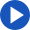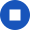# sum

Change speakerYou're listening to Joanna
She has an American accent.

•••••NGSL Rank: 1372
sum
sʌmMeanings
noun
• a particular amount of money.
E.g. they could not afford such a sum
• the total amount resulting from the addition of two or more numbers, amounts, or items.
E.g. the sum of two prime numbers
• an arithmetical problem, especially at an elementary level.
E.g. we did sums at school, Mummy
verb
• find the sum of (two or more amounts).
E.g. if we sum these equations we obtain X

### Practise saying this word

sum
JoannaYouReset